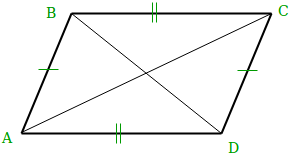# Find all possible coordinates of parallelogram

Find the all the possible coordinate from the given three coordinates to make a parallelogram of a non-zero area.
Let’s call A,B,C are the three given points. We can have only the three possible situations:

```(1) AB and AC are sides, and BC a diagonal
(2) AB and BC are sides, and AC a diagonal
(3) BC and AC are sides, and AB a diagonal ```

Hence, we can say that only 3 coordinates are possible from which we can generate a parallelogram if three coordinates are given.
To prove that all the three points are different let’s suppose it’s wrong. Without losing of generality suppose that the points got in cases AD and BC are equal.

Consider the system of two equations for the equality of these points:

```Bx + Cx - Ax = Ax + Cx - Bx
By + Cy - Ay = Ay + Cy - By

It can be simplified as-

Ax = Bx
Ay = By```

And we got a contradiction, as all the points A, B, C are distinct.

Examples:

```Input  : A = (0 0)
B = (1 0)
C = (0 1)
Output :  1 -1
-1 1
1 1

Input  : A = (-1 -1)
B = (0 1)
C = (1 1)
Output : -2 -1
0 -1
2 3```Since the opposite sides are equal, AD = BC and AB = CD, we can calculate the co-ordinates of the missing point (D) as:

```AD = BC
(Dx - Ax, Dy - Ay) = (Cx - Bx, Cy - By)
Dx = Ax + Cx - Bx
Dy = Ay + Cy - By```

The cases where the diagonals are AD and BC, CD and AB are processed in the same way.
Reference: https://math.stackexchange.com/questions/1322535/how-many-different-parallelograms-can-be-drawn-if-given-three-co-ordinates-in-3d

Below is the implementation of above approach:

## C++

 `// C++ program to all possible points` `// of a parallelogram` `#include ` `using` `namespace` `std;` ` `  `// main method` `int` `main()` `{` `   ``int` `ax = 5, ay = 0; ``//coordinates of A` `   ``int` `bx = 1, by = 1; ``//coordinates of B` `   ``int` `cx = 2, cy = 5; ``//coordinates of C` `    ``cout << ax + bx - cx << ``", "` `         ``<< ay + by - cy <

## Java

 `// Java program to all possible ` `// points of a parallelogram` `public` `class` `ParallelogramPoints{ ` `    `  `    ``// Driver code` `    ``public` `static` `void` `main(String[] s)` `    ``{` `        ``int` `ax = ``5``, ay = ``0``; ``//coordinates of A` `        ``int` `bx = ``1``, by = ``1``; ``//coordinates of B` `        ``int` `cx = ``2``, cy = ``5``; ``//coordinates of C` `        ``System.out.println(ax + bx - cx + ``", "` `                           ``+ (ay + by - cy));` `        ``System.out.println(ax + cx - bx + ``", "` `                           ``+ (ay + cy - by));` `        ``System.out.println(cx + bx - ax + ``", "` `                           ``+ (cy + by - ax));` `    ``}` `}`   `// This code is contributed by Prerna Saini`

## Python3

 `# Python3 program to find all possible points` `# of a parallelogram`   `ax ``=` `5` `ay ``=` `0` `#coordinates of A` `bx ``=` `1` `by ``=` `1` `#coordinates of B` `cx ``=` `2` `cy ``=` `5` `#coordinates of C` `print``(ax ``+` `bx ``-` `cx, ``", "``, ay ``+` `by ``-` `cy)` `print``(ax ``+` `cx ``-` `bx, ``", "``, ay ``+` `cy ``-` `by)` `print``(cx ``+` `bx ``-` `ax, ``", "``, cy ``+` `by ``-` `ax)`

## C#

 `// C# program to all possible ` `// points of a parallelogram` `using` `System;`   `public` `class` `ParallelogramPoints` `{ ` `    `  `    ``// Driver code` `    ``public` `static` `void` `Main()` `    ``{` `        `  `        ``//coordinates of A` `        ``int` `ax = 5, ay = 0; ` `        `  `        ``//coordinates of B` `        ``int` `bx = 1, ``by` `= 1; ` `        `  `        ``//coordinates of C` `        ``int` `cx = 2, cy = 5; ` `        `  `        ``Console.WriteLine(ax + bx - cx + ``", "` `                        ``+ (ay + ``by` `- cy));` `        ``Console.WriteLine(ax + cx - bx + ``", "` `                        ``+ (ay + cy - ``by``));` `        ``Console.WriteLine(cx + bx - ax + ``", "` `                        ``+ (cy + ``by` `- ax));` `    ``}` `}`   `// This code is contributed by vt_m.`

## PHP

 ``

## Javascript

 ``

Output:

```4, -4
6, 4
-2, 1```

Time Complexity: O(1)
Auxiliary Space: O(1)

Please suggest if someone has a better solution which is more efficient in terms of space and time.